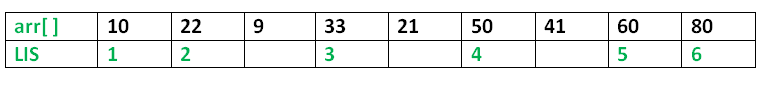# C/C++ Program for Longest Increasing Subsequence

• Difficulty Level : Easy
• Last Updated : 01 Jun, 2021

The Longest Increasing Subsequence (LIS) problem is to find the length of the longest subsequence of a given sequence such that all elements of the subsequence are sorted in increasing order. For example, the length of LIS for {10, 22, 9, 33, 21, 50, 41, 60, 80} is 6 and LIS is {10, 22, 33, 50, 60, 80}.More Examples:

```Input  : arr[] = {3, 10, 2, 1, 20}
Output : Length of LIS = 3
The longest increasing subsequence is 3, 10, 20

Input  : arr[] = {3, 2}
Output : Length of LIS = 1
The longest increasing subsequences are {3} and {2}

Input : arr[] = {50, 3, 10, 7, 40, 80}
Output : Length of LIS = 4
The longest increasing subsequence is {3, 7, 40, 80}```

Optimal Substructure:
Let arr[0..n-1] be the input array and L(i) be the length of the LIS ending at index i such that arr[i] is the last element of the LIS.
Then, L(i) can be recursively written as:
L(i) = 1 + max( L(j) ) where 0 < j < i and arr[j] < arr[i]; or
L(i) = 1, if no such j exists.
To find the LIS for a given array, we need to return max(L(i)) where 0 < i < n.
Thus, we see the LIS problem satisfies the optimal substructure property as the main problem can be solved using solutions to subproblems.

Following is a simple recursive implementation of the LIS problem. It follows the recursive structure discussed above.

## C++

 `/* A Naive C++ recursive implementation of LIS problem */``#include ``using` `namespace` `std;` `/* To make use of recursive calls, this function must return``   ``two things:``   ``1) Length of LIS ending with element arr[n-1]. We use``      ``max_ending_here for this purpose``   ``2) Overall maximum as the LIS may end with an element``      ``before arr[n-1] max_ref is used this purpose.``   ``The value of LIS of full array of size n is stored in``   ``*max_ref which is our final result */``int` `_lis(``int` `arr[], ``int` `n, ``int``* max_ref)``{``    ` `    ``/* Base case */``    ``if` `(n == 1)``        ``return` `1;` `    ``// 'max_ending_here' is length of LIS ending with arr[n-1]``    ``int` `res, max_ending_here = 1;` `    ``/* Recursively get all LIS ending with arr, arr ...``       ``arr[n-2]. If   arr[i-1] is smaller than arr[n-1], and``       ``max ending with arr[n-1] needs to be updated, then``       ``update it */``    ``for``(``int` `i = 1; i < n; i++)``    ``{``        ``res = _lis(arr, i, max_ref);``        ``if` `(arr[i - 1] < arr[n - 1] &&``              ``res + 1 > max_ending_here)``            ``max_ending_here = res + 1;``    ``}` `    ``// Compare max_ending_here with the overall max. And``    ``// update the overall max if needed``    ``if` `(*max_ref < max_ending_here)``        ``*max_ref = max_ending_here;` `    ``// Return length of LIS ending with arr[n-1]``    ``return` `max_ending_here;``}` `// The wrapper function for _lis()``int` `lis(``int` `arr[], ``int` `n)``{``    ` `    ``// The max variable holds the result``    ``int` `max = 1;` `    ``// The function _lis() stores its result in max``    ``_lis(arr, n, &max);` `    ``// returns max``    ``return` `max;``}` `// Driver code``int` `main()``{``    ``int` `arr[] = { 10, 22, 9, 33, 21, 50, 41, 60 };``    ``int` `n = ``sizeof``(arr) / ``sizeof``(arr);``    ` `    ``cout << ``"Length of lis is "` `<< lis(arr, n) << ``"\n"``;``    ``return` `0;``}` `// This code is contributed by Shubhamsingh10`

## C

 `/* A Naive C recursive implementation of LIS problem */``#include ``#include ` `/* To make use of recursive calls, this function must return``   ``two things:``   ``1) Length of LIS ending with element arr[n-1]. We use``      ``max_ending_here for this purpose``   ``2) Overall maximum as the LIS may end with an element``      ``before arr[n-1] max_ref is used this purpose.``   ``The value of LIS of full array of size n is stored in``   ``*max_ref which is our final result */``int` `_lis(``int` `arr[], ``int` `n, ``int``* max_ref)``{``    ``/* Base case */``    ``if` `(n == 1)``        ``return` `1;` `    ``// 'max_ending_here' is length of LIS ending with arr[n-1]``    ``int` `res, max_ending_here = 1;` `    ``/* Recursively get all LIS ending with arr, arr ...``       ``arr[n-2]. If   arr[i-1] is smaller than arr[n-1], and``       ``max ending with arr[n-1] needs to be updated, then``       ``update it */``    ``for` `(``int` `i = 1; i < n; i++) {``        ``res = _lis(arr, i, max_ref);``        ``if` `(arr[i - 1] < arr[n - 1] && res + 1 > max_ending_here)``            ``max_ending_here = res + 1;``    ``}` `    ``// Compare max_ending_here with the overall max. And``    ``// update the overall max if needed``    ``if` `(*max_ref < max_ending_here)``        ``*max_ref = max_ending_here;` `    ``// Return length of LIS ending with arr[n-1]``    ``return` `max_ending_here;``}` `// The wrapper function for _lis()``int` `lis(``int` `arr[], ``int` `n)``{``    ``// The max variable holds the result``    ``int` `max = 1;` `    ``// The function _lis() stores its result in max``    ``_lis(arr, n, &max);` `    ``// returns max``    ``return` `max;``}` `/* Driver program to test above function */``int` `main()``{``    ``int` `arr[] = { 10, 22, 9, 33, 21, 50, 41, 60 };``    ``int` `n = ``sizeof``(arr) / ``sizeof``(arr);``    ``printf``(``"Length of lis is %d\n"``,``           ``lis(arr, n));``    ``return` `0;``}`
Output:
`Length of lis is 5`

Please refer complete article on Dynamic Programming | Set 3 (Longest Increasing Subsequence) for more details!

My Personal Notes arrow_drop_up